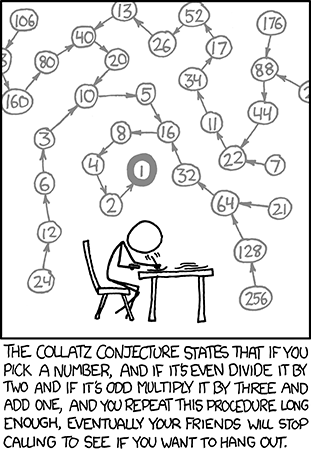# The simplest unsolved math problem

## Exploring math with my 8 year old niece

Posted by Janne Cederberg on Jun. 25, 2016
Categories: Mathematics

## Background

Yesterday with my niece we came across an interesting YouTube video about what the video claims to be the simplest unsolved (they use the word impossible) math problem:

In layman terms the problem is defined as follows:

1. Pick any positive whole number.
2. If the number is even, divide it by 2. If it was odd however, multiply it by 3 and add 1.
3. Repeat step 2 infinitely (or as hypothesized until you reach value 1, which should/seems to always happen).

Mathematically the same sequence is produced by this recursive definition:

$$a_n = \begin{cases} \text{Select any } & a_1\in\mathbb{N} \\ \frac{a_{n-1}}{2} & \text{if } n > 1 \text{ and } a_{n-1} \text{ is even} \\ 3a_{n-1}+1 & \text{if } n > 1 \text{ and } a_{n-1} \text{ is odd} \end{cases}$$

The claim is that the series formed by the aforementioned recursive definition will eventually always reach 1. This is also known as the Collatz Conjecture.

## Exploring the problem

The problem is quite readily understandable for a primary school student; my niece is 8 years old at the moment and she seemed fashinated by it. We worked though the sequence starting from 10 and from 100 and reached 1 with both starting points.

Today to amuse myself and my niece, I created the following illustration:

## Try it yourself

Alter the sequence start value either by up/down arrow keys on the keyboard or inputting a value and pressing enter.

Sequence start:
Term count:
Largest term:

## Conclusion

My niece seemed to enjoy exploring this. She was surprised by the fact that mathematicians haven’t been able to figure out why this works, e.g. that whatever positive whole number that you choose as your starting point, you’ll eventually reach 1 no matter what.

For her this was a though-experiment and some motivation regarding multiplication/division. For me it was a small quick-and-dirty data-illustration exercise :)

## Humor segment

xkcd has also covered the Collatz conjecture with an ironic twist: